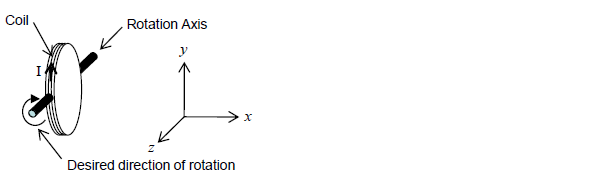# Problem: The coil in the figure below lies in the y-z plane, has a current, I, in the direction shown and is free to rotate about the z-axis. What should the direction of an external B-field be to cause a clock-wise rotation as shown? A. Positive y-direction B. Negative y-direction C. Positive z-direction D. Negative z-direction E. Negative x-direction

###### Problem Details

The coil in the figure below lies in the y-z plane, has a current, I, in the direction shown and is free to rotate about the z-axis. What should the direction of an external B-field be to cause a clock-wise rotation as shown?

A. Positive y-direction

B. Negative y-direction

C. Positive z-direction

D. Negative z-direction

E. Negative x-direction